## 6.4.1 YSZ Deposition using ESD Pyrolysis

The YSZ deposition using an ESD process from  is simulated using the model discussed in Section 5.1. The parameters of the solution used and therefore, the properties of the droplets which are simulated are listed in Table 6.1. The first step is finding the droplet size as they exit the atomizer. The distribution of droplet sizes does not follow any standard distribution, but it is suggested that the volume fraction varies relatively evenly near the approximate value 0.05 for droplets between with a radius between 2.5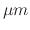and 55, . Therefore, the distribution for the droplet radii is simulated by generating an even distribution for the volume fraction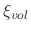followed by finding the radius distribution for the droplet. Droplets with a radius below 2.5are ignored, because they do not take part in the deposition reaction . The distribution of the droplet's radius is derived in app:radius and given by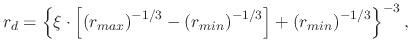(204)

where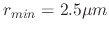and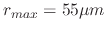are the minimum and maximum radii for the generated droplets and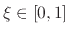is an evenly distributed random value.

As soon as a droplet is generated and exits the atomizer, it experiences a strong electric force, much stronger than the gravitational force. This initial push from the atomizer determines the droplet's speed and direction as it enters the electric field. Because there is a very quick drop in the electric field magnitude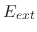from (5.20), the initial calculation is done within a very small radius of the atomizing nozzle (0.005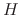). The distribution of the initial starting position of particles will take into account the droplet distribution angle of the nozzle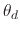and will be found using spherical coordinates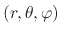.

The given equation for the electric field provides the magnitude at each location; however, in order to follow the droplet trajectory, the individual components of the electric field in each direction are required such that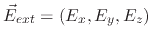. The individual components of the electric field in each Cartesian direction can be found by equating a unit vector in the form similar to (3.35). However, since the effects of the forces in the horizontal directions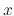andare equal, calculations are easier using cylindrical coordinates, where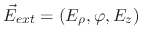. The droplet angle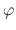is unaffected by the applied forces since they act only in the radial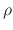and vertical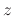directions. Therefore, the initial starting position's spherical coordinates will be converted to cylindrical coordinates, which will remain until the trajectory is complete.

Figure 6.16 shows a silicon surface geometry which extends 50mm by 50mm after 1, 10, 20, 50, and 100 spray cycles with 100,000 droplets per cycle and the spray nozzle located 270mm above the surface.

Figure 6.16: Macroscopic spray pyrolysis simulation on a 50mm by 50mm geometry. Each spray cycle contains 100,000 droplets.(a) Initial geometry (b) After 1 spray cycle (c) After 10 spray cycles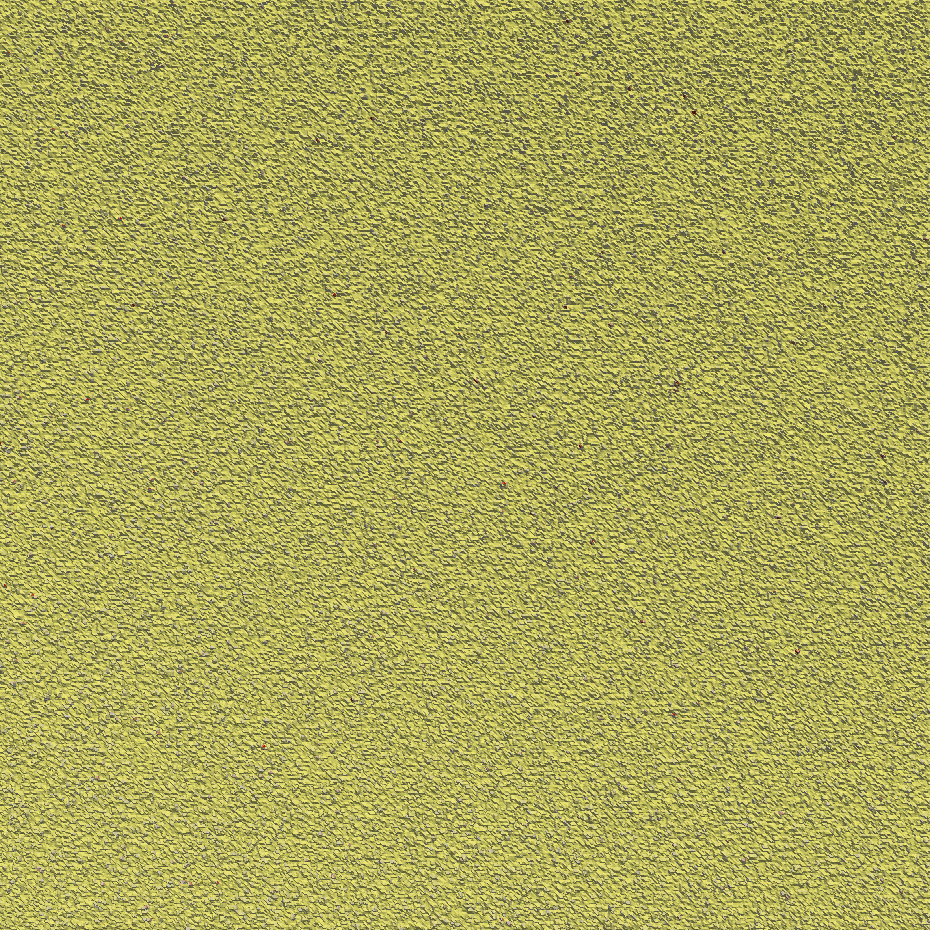(d) After 20 spray cycles (e) After 50 spray cycles (f) After 100 spray cycles

The images in Figure 6.16 show little in the way the thin film is deposited when the deposition process is modeled as a sequence of droplets landing onto the surface and depositing a disk shaped film. Figure 6.17 shows an area which expands 250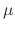m by 250m. Several droplets are shown including overlapping of the disk shapes on the surface as they are being deposited. The yellow surface is silicon while the orange disks are the deposited YSZ films. Each depositing droplet is modeled using 10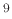particles which accelerate to the surface and add a slight component of the overall deposited film thickness.

Figure 6.17: Microscopic spray pyrolysis simulation on a 250m by 250m geometry.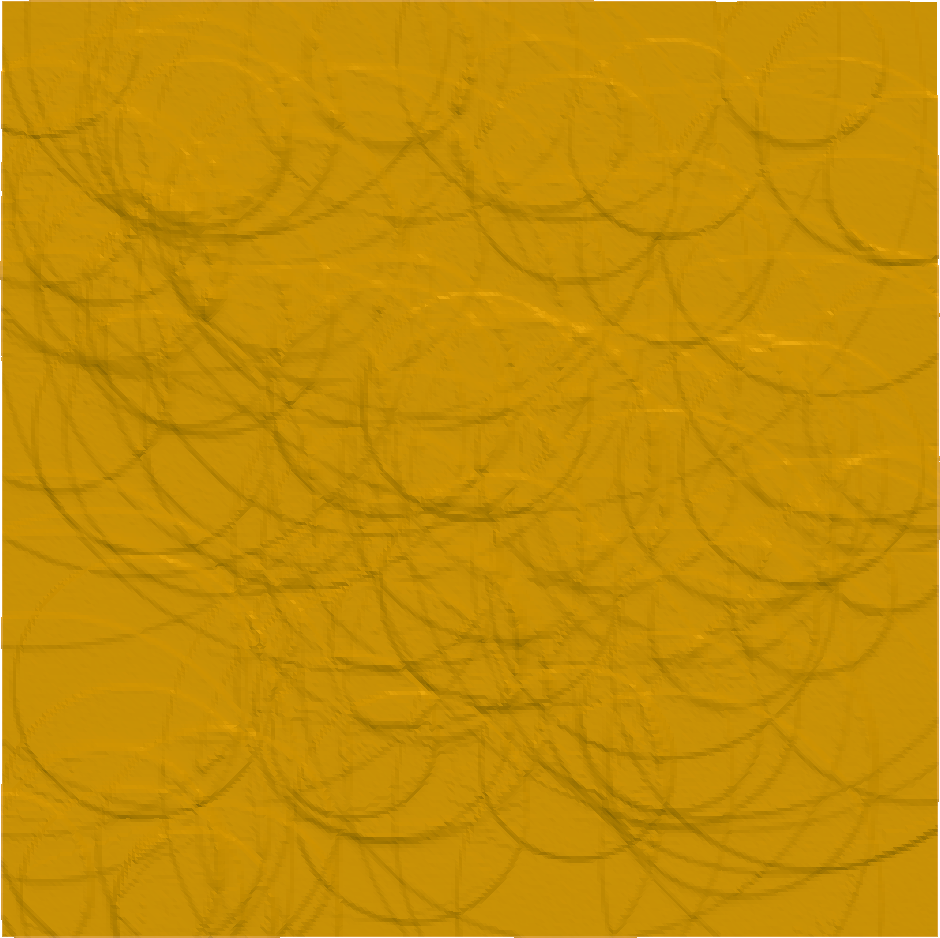(a) 15 droplets (b) 100 droplets

L. Filipovic: Topography Simulation of Novel Processing Techniques